# JP2007208423A - Analog to digital converter - Google Patents

## Info

Publication number
JP2007208423A
JP2007208423A JP2006022494A JP2006022494A JP2007208423A JP 2007208423 A JP2007208423 A JP 2007208423A JP 2006022494 A JP2006022494 A JP 2006022494A JP 2006022494 A JP2006022494 A JP 2006022494A JP 2007208423 A JP2007208423 A JP 2007208423A
Authority
JP
Japan
Prior art keywords
encoder
analog
input
analog signal
circuit
Prior art date
Legal status (The legal status is an assumption and is not a legal conclusion. Google has not performed a legal analysis and makes no representation as to the accuracy of the status listed.)
Pending
Application number
JP2006022494A
Other languages
Japanese (ja)
Inventor
Shigeto Kobayashi

Original Assignee
Sanyo Electric Co Ltd

Priority date (The priority date is an assumption and is not a legal conclusion. Google has not performed a legal analysis and makes no representation as to the accuracy of the date listed.)
Filing date
Publication date
Application filed by Sanyo Electric Co Ltd, 三洋電機株式会社 filed Critical Sanyo Electric Co Ltd
Priority to JP2006022494A priority Critical patent/JP2007208423A/en
Publication of JP2007208423A publication Critical patent/JP2007208423A/en
Pending legal-status Critical Current

• 230000000875 corresponding Effects 0 claims description 2
• 238000006243 chemical reaction Methods 0 description 48
• 238000000034 methods Methods 0 description 7
• 230000000694 effects Effects 0 description 3

## Images

•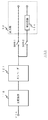•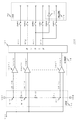•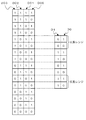•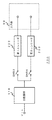•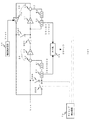•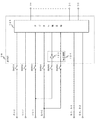••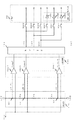•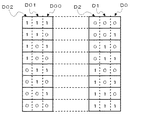## Classifications

• HELECTRICITY
• H03BASIC ELECTRONIC CIRCUITRY
• H03MCODING; DECODING; CODE CONVERSION IN GENERAL
• H03M1/00Analogue/digital conversion; Digital/analogue conversion
• H03M1/004Reconfigurable analogue/digital or digital/analogue converters
• H03M1/007Reconfigurable analogue/digital or digital/analogue converters among different resolutions
• HELECTRICITY
• H03BASIC ELECTRONIC CIRCUITRY
• H03MCODING; DECODING; CODE CONVERSION IN GENERAL
• H03M1/00Analogue/digital conversion; Digital/analogue conversion
• H03M1/12Analogue/digital converters
• H03M1/14Conversion in steps with each step involving the same or a different conversion means and delivering more than one bit
• H03M1/16Conversion in steps with each step involving the same or a different conversion means and delivering more than one bit with scale factor modification, i.e. by changing the amplification between the steps
• H03M1/162Conversion in steps with each step involving the same or a different conversion means and delivering more than one bit with scale factor modification, i.e. by changing the amplification between the steps the steps being performed sequentially in a single stage, i.e. recirculation type
• HELECTRICITY
• H03BASIC ELECTRONIC CIRCUITRY
• H03MCODING; DECODING; CODE CONVERSION IN GENERAL
• H03M1/00Analogue/digital conversion; Digital/analogue conversion
• H03M1/12Analogue/digital converters
• H03M1/14Conversion in steps with each step involving the same or a different conversion means and delivering more than one bit
• H03M1/16Conversion in steps with each step involving the same or a different conversion means and delivering more than one bit with scale factor modification, i.e. by changing the amplification between the steps
• H03M1/164Conversion in steps with each step involving the same or a different conversion means and delivering more than one bit with scale factor modification, i.e. by changing the amplification between the steps the steps being performed sequentially in series-connected stages
• H03M1/167Conversion in steps with each step involving the same or a different conversion means and delivering more than one bit with scale factor modification, i.e. by changing the amplification between the steps the steps being performed sequentially in series-connected stages all stages comprising simultaneous converters

## Abstract

<P>PROBLEM TO BE SOLVED: To provide an analog to digital converter the circuit scale of which is reduced. <P>SOLUTION: The analog to digital converter 100 includes: a resistor group 116; a comparator group 118; an encoder 121; and an output section 98. The output section 98 includes a correction circuit 124. The correction circuit 124 comprises an inverter 126. The encoder 121 is shared between the case when the analog to digital converter 100 converts a received analog signal Vin into a 4-bit binary code and the case when the analog to digital converter 100 converts the received analog signal Vin into a 2-bit binary code. The correction circuit 124 corrects the output of the encoder 121 when the analog to digital converter 100 is to convert the received analog signal Vin into the 2-bit binary code. <P>COPYRIGHT: (C)2007,JPO&INPIT

## Description

The present invention relates to an analog-digital converter, and more particularly to an analog-digital converter that compares an input analog signal with a reference voltage using a plurality of comparators provided in parallel.

As an example of a circuit for converting an input analog signal into a digital signal, there is a pipeline AD converter (see, for example, Patent Document 1). The pipeline type AD converter is configured by connecting a plurality of low bit sub AD converters. The input analog signal is AD-converted step by step by each sub AD converter. The sub AD converter includes a plurality of comparators, and converts the analog signal into a digital signal by comparing the input analog signal with a reference voltage.
Japanese Patent Laid-Open No. 9-275342

A digital signal obtained by comparing the input analog signal with a reference voltage is converted into a binary code by an encoder. The configuration of the encoder is determined according to the number of bits of the binary code to be generated. That is, if the number of bits of the binary code to be generated is different, the configuration of the encoder is also different. Therefore, for example, when a binary code having two different numbers of bits is generated, even if a circuit that compares an input analog signal with a reference voltage is shared, it is necessary to prepare two encoders. This hinders reduction in the circuit scale of the analog-digital converter.

The present invention has been made in view of such a situation, and an object thereof is to reduce the circuit scale of an analog-digital converter.

In order to solve the above-described problem, an analog-digital converter according to an aspect of the present invention is an analog-digital converter that converts an input analog signal into a digital value having a predetermined number of bits. Multiple comparators that compare with a given reference voltage, encoders that convert the outputs of multiple comparators into digital values, and corrections that correct the encoder output as needed depending on the circumstances in which the analog-to-digital converter is used Circuit.

The “situation to be used” is determined by, for example, the number of bits of a digital value to be generated by the analog-digital converter or the specification of the input of a signal to the comparator. According to this aspect, since the correction circuit is provided, the encoder can be shared even if the situation in which the analog-digital converter is used differs, so that the circuit scale of the analog-digital converter can be reduced.

Another aspect of the present invention is also an analog-digital converter. The analog-to-digital converter is an analog-to-digital converter that converts an input analog signal into a digital value having a first number of bits, and is configured by arranging a number of comparators determined by the first number of bits in parallel. A comparator group for comparing the input analog signal with a predetermined reference voltage, an encoder for converting the output of the comparator group into a digital value of the first number of bits, and a digital value converted by the encoder is output. And an output unit. The output unit converts the digital value converted by the encoder into an analog-to-digital converter when the analog signal input by the analog-to-digital converter is to be converted into a digital value having a second number of bits smaller than the first number of bits. Are corrected to a digital value of the second number of bits to be generated and output.

According to this aspect, since the output unit has a function of correcting the digital value converted by the encoder, in the conversion of the first number of bits into the digital value and the conversion of the second number of bits into the digital value, the encoder is Can be shared. Thereby, the circuit scale of the analog-digital converter can be reduced.

The first bit number is 4, the second bit number is 2, and the output unit may include an inverter that inverts the value of the second bit from the lower order of the digital values converted by the encoder. The output of the inverter and the value of the least significant bit of the digital value converted by the encoder may be output as a digital value of the second number of bits to be generated by the analog-digital converter. In this case, the configuration for correcting the digital value of the second number of bits to be generated by the analog-to-digital converter can be realized by one inverter, so that the circuit scale of the analog-to-digital converter can be reduced.

Yet another embodiment of the present invention is also an analog-digital converter. The analog-digital converter includes a plurality of comparators that compare an input analog signal with a predetermined reference voltage, and an analog signal and a predetermined reference voltage that are input to each of the plurality of comparators according to a first specification. An encoder configured to correspond to the case of input and converting the outputs of a plurality of comparators into digital values, and an analog signal input to each of the plurality of comparators according to a second specification different from the first specification And a correction circuit that corrects the digital value converted by the encoder when a predetermined reference voltage is input.

According to this aspect, by providing the correction circuit, the encoder can be shared when the specifications of the input of the analog signal and the reference voltage for each of the plurality of comparators are different, so the circuit scale of the analog-digital converter can be reduced. Can be reduced.

A plurality of comparators are used when an analog signal and a predetermined reference voltage input according to the first specification are input and when an analog signal and a predetermined reference voltage input according to the second specification are input. The magnitude relationship between the output levels in each of the above may be reversed. The correction circuit may include an inverter that inverts the digital value converted by the encoder. In this case, since the correction circuit can be realized with a simple configuration, the circuit scale of the analog-digital converter can be reduced.

According to the present invention, the circuit scale of the analog-digital converter can be reduced.

The embodiment relates to an analog-digital converter (hereinafter referred to as “AD converter”) that converts an input analog signal into a digital value by comparing it with a reference voltage. This AD converter is basically configured to convert an input analog signal into a digital value having a first number of bits. When the analog signal to which the AD converter is input is to be converted into a digital value having a second bit number smaller than the first bit number, the first obtained from the encoder as a result of comparison with the reference voltage The digital value of the number of bits is corrected to the digital value of the second number of bits to be generated by the AD converter. Thus, the analog signal input to the AD converter should be converted to a digital value having the first number of bits, and the analog signal input to the AD converter should be converted to a digital value having the second number of bits. In some cases, the encoder can be shared. Therefore, the circuit scale of the AD converter can be reduced as compared with the case where a separate encoder is provided for each of the above two cases.

(First embodiment)
FIG. 1 shows a schematic configuration of an AD converter 100 according to the first embodiment. The AD converter 100 is configured on the assumption that an input analog signal is converted into an n-bit binary code. The schematic configuration of the AD converter 100 includes a comparator group 118, an encoder 121, and an output unit 98. The output unit 98 includes a correction circuit 124.

The comparator group 118 includes a plurality of comparators arranged in parallel. The comparator group 118 compares the input analog signal with the reference voltage and outputs the comparison result to the encoder 121. The encoder 121 converts the comparison result in the comparator group 118 into an n-bit binary code. The encoder 121 is configured by, for example, a ROM (Read Only Memory). The n-bit binary code output from the encoder 121 is output to the output unit 98 via the switch SW91 or the switch SW92. The switch SW91 and the switch SW92 are exclusively turned on / off.

The switch SW91 is turned on when the analog signal Vin input to the AD converter 100 is to be converted into an n-bit binary code. The switch SW92 is turned on when the analog signal Vin input to the AD converter 100 is to be converted into a binary code of m bits (m satisfies m <n). The switch SW 92 may input only a portion to be used in the correction circuit 124 of the n-bit binary code output from the encoder 121 to the correction circuit 124.

The output unit 98 outputs the n-bit binary code input via the switch SW91 as it is. On the other hand, the output unit 98 corrects the binary code input via the SW 92 into an m-bit binary code to be generated by the AD converter 100 in the correction circuit 124 and outputs the m-bit binary code. Hereinafter, description will be made assuming that n = 4 and m = 2.

FIG. 2 shows the overall configuration of the AD converter 100 of FIG. The AD converter 100 shown in FIG. 2 is a fully parallel comparison, that is, a flash method. In FIG. 2, the same or similar components as those in FIG. In FIG. 2, the analog signal Vin and the reference voltage show only one of the differential inputs, and the other is not shown.

The AD converter 100 includes a resistance group 116, a comparator group 118, an encoder 121, and an output unit 98. The resistor group 116 includes 14 resistors R1 to R14 connected in series between the terminal of the high potential side reference voltage VRT and the terminal of the low potential side reference voltage VRB. The comparator group 118 includes 15 comparators Cmp1 to Cmp15 arranged in parallel. The output unit 98 includes a correction circuit 124. The correction circuit 124 includes an inverter 126.

The nodes on the low potential side of the resistors R1 to R14 are referred to as nodes N1 to N14. A node on the high potential side of the resistor R14 is referred to as a node N15. The potentials VR (1) to VR (15) of the nodes N1 to N15 serve as reference voltages for the comparators Cmp1 to Cmp15. Each of the resistors R1 to R14 has the same resistance value. Therefore, the reference voltages VR (1) to VR (15) are 15 reference voltages having equal potential intervals.

The analog signal Vin is input to the non-inverting input terminals of the comparators Cmp1 to Cmp15. Reference voltages VR (1) to VR (15), which are potentials of the nodes N1 to N15, are input to the inverting input terminals of the comparators Cmp1 to Cmp15, respectively. Therefore, each of the output signals Vcmp1 to Vcmp15 of the comparators Cmp1 to Cmp15 is at a high level when the analog signal Vin is larger than each of the reference voltages VR (1) to VR (15). Conversely, the output signals Vcmp1 to Vcmp15 of the comparators Cmp1 to Cmp15 are at a low level when the analog signal Vin is smaller than each of the reference voltages VR (1) to VR (15).

Outputs Vcmp1 to Vcmp15 of the comparators Cmp1 to Cmp15 are input to the encoder 121. The encoder 121 converts the input signal into a 4-bit binary code (D03 to D00). The 4-bit binary code (D03 to D00) output from the encoder 121 is output to the output unit 98 via the switches SW81 to SW84. Alternatively, the lower 2 bits (D01 to D00) of the 4-bit binary code (D03 to D00) output from the encoder 121 are output to the output unit 98 via the switches SW85 and SW86.

The switches SW81 to SW84 correspond to the switch SW91 in FIG. The switch SW85 and the switch SW86 correspond to the switch SW92 in FIG. When the AD converter 100 is to convert the input analog signal Vin into a 4-bit binary code, the switches SW81 to SW84 are turned on, and the SW85 and the switch SW86 are turned off. When the AD converter 100 is to convert the input analog signal Vin into a 2-bit binary code, the SW85 and the switch SW86 are turned on, and the switches SW81 to SW84 are turned off.

The output unit 98 outputs the 4-bit binary code (D03 to D00) input via the switches SW81 to SW84 as it is. On the other hand, the output unit 98 corrects the lower 2-bit binary code (D01 to D00) input via the switch SW85 and the switch SW86 and outputs the corrected binary code (D01 to D00). In the case of FIG. 2, the correction circuit 124 includes an inverter 126 connected to the switch SW85. Therefore, the output unit 98 inverts and outputs the binary code (D01) input via the switch SW85, and outputs the binary code (D00) input via the switch SW86 as it is.

The correction in the correction circuit 124 will be described. 3 shows a 4-bit binary code (D03 to D00) output from the encoder 121 of FIG. 2 and the 2-bit binary code when the AD converter 100 of FIG. 2 should generate a 2-bit binary code. The correspondence relationship with (D1-D0) is shown. In FIG. 3, the lowest D03 to D00 are the outputs of the encoder 121 when the outputs of all the comparators in the comparator group 118 of FIG. 2 are at a low level. D03 to D00 of the i-th (2 <i <15) from the bottom are the outputs of the comparators Vcmp1 to Vcmp (i-1) in the comparator group 118 of FIG. This is the output of the encoder 121 when the output is at a low level. D03 to D00 at the top are the outputs of the encoder 121 when the outputs of all the comparators in the comparator group 118 in FIG. 2 are at a high level.

In this embodiment, when the analog signal Vin input to the AD converter 100 in FIG. 2 is to be converted into a 2-bit binary code, the comparators Vcmp5 to Vcmp11 in the comparator group 118 in FIG. 2 are used. Therefore, from the bottom to the fifth to twelfth of the fourteen 4-bit binary codes (D03 to D00) shown in FIG. 3, the correction circuit 124 of FIG.

Referring to FIG. 3, D00 of the 4-bit binary code (D03 to D00) output from the encoder 121 is the same as the 2-bit binary code (D1 to D0) to be generated by the AD converter 100. It can be seen that it may be output as D0. If D01 of the 4-bit binary code (D03 to D00) output from the encoder 121 is inverted, D1 of the 2-bit binary code (D1 to D0) to be generated by the AD converter 100 is obtained. You can see that Therefore, as shown in FIG. 2, the correction circuit 124 can be configured by only the inverter 126 provided on the path D01 output from the encoder 121. Of the D1 to D0 shown in FIG. 3, two from the top and two from the bottom are redundant ranges. The redundant range is provided for correcting the conversion result of the upper bits when the configuration shown in FIG. 2 is included in, for example, a pipeline type AD converter. When such correction is unnecessary, it is not necessary to provide a redundant range.

According to the present embodiment, by providing the correction circuit 124 in the output unit 98, the AD converter 100 converts the input analog signal Vin into a 4-bit binary code, and the AD converter 100 The encoder 121 can be shared when the input analog signal Vin is to be converted into a 2-bit binary code. Further, as shown in FIG. 2, the correction circuit 124 can be configured by only an inverter 126, for example. Therefore, the circuit scale of the AD converter 100 can be reduced as compared with the case where a separate encoder is provided for each of the above two cases.

In order to clarify the effect of the present embodiment, an AD converter provided with two encoders for generating binary codes having two different types of bits will be briefly described. FIG. 4 shows a schematic configuration of the AD converter 200 according to the comparative example. Unlike the configuration of FIG. 1, the AD converter 200 includes a first encoder 222 for generating a 4-bit binary code and a second encoder 223 for generating a 2-bit binary code. . On the other hand, the output unit 99 is not provided with a correction circuit. The second encoder 223 requires a larger circuit scale than the correction circuit 124 of FIG. In the configuration of FIG. 2, the correction circuit 124 can be configured by only the inverter 126, and thus the effect of reducing the circuit scale according to the present embodiment is obvious.

(Second Embodiment)
In the second embodiment, the configuration of the first embodiment shown in FIG. 2 is mounted on an AD converter that divides an input analog signal into a plurality of times and converts it into a digital value step by step. Will be explained. FIG. 5 shows a configuration of the AD converter 101 according to the second embodiment. In FIG. 5, the same or similar components as those in FIG.

The AD converter 101 includes a third switch SW3, a fourth switch SW4, a second AD conversion circuit 20, a second DA conversion circuit 21, a second subtraction circuit 22, a third amplification circuit 23, and a first switch SW1. The second switch SW2, the first amplifier circuit 11, the first AD converter circuit 92, the first DA converter circuit 93, the first subtractor circuit 94, the second amplifier circuit 15, the timing control circuit 18, and the amplifier. A control circuit 19 and an output unit 98 are provided. The resistor group 116, the comparator group 118, and the encoder 121 in the configuration illustrated in FIG. 2 are included in the second AD conversion circuit 20.

In the AD converter 101, the analog signal Vin is input to the second AD conversion circuit 20 via the fourth switch SW4. The second AD conversion circuit 20 compares the input analog signal with the reference voltage, and outputs a digital value as a comparison result to the second DA conversion circuit 21. Further, the second AD conversion circuit 20 converts the digital value of the comparison result into a 4-bit binary code and outputs it to the output unit 98. The second DA conversion circuit 21 converts the digital value output from the second AD conversion circuit 20 into an analog signal.

The second subtraction circuit 22 subtracts the output of the second DA conversion circuit 21 from the analog signal Vin. The third amplification circuit 23 amplifies the output of the second subtraction circuit 22. The output of the third amplifier circuit 23 is output to the first amplifier circuit 11 and the first AD converter circuit 92 via the first switch SW1. The amplification factor of the third amplifier circuit 23 is twice. Instead of the second subtraction circuit 22 and the third amplification circuit 23, a second subtraction amplification circuit 24 that is an amplification circuit having a subtraction function may be used. A sample hold circuit may be inserted before the second subtracting circuit 22. In the example of FIG. 5, subtraction is performed by adjusting the input timing to the second subtraction amplification circuit 24.

The first AD conversion circuit 92 compares the input analog signal with the reference voltage, and outputs a digital value of the comparison result to the first DA conversion circuit 93. The first AD conversion circuit 92 converts the digital value of the comparison result into a 2-bit binary code and outputs it to the output unit 98. The first DA conversion circuit 93 converts the digital value output from the first AD conversion circuit 92 into an analog signal. Here, the analog value output from the first DA converter circuit 93 is amplified by a factor of 2 corresponding to the amplification factor of the first amplifier circuit 11.

The first amplifier circuit 11 samples and holds the input analog signal, amplifies it twice, and outputs it to the first subtraction circuit 94. The first subtraction circuit 94 subtracts the analog value output from the first DA conversion circuit 93 from the analog value output from the first amplifier circuit 11. The second amplifier circuit 15 amplifies the output of the first subtraction circuit 94. The amplification factor of the second amplifier circuit 15 is controlled by an amplification control signal Vcnt output from the amplification control circuit 19. The output of the second amplifier circuit 15 is fed back to the first amplifier circuit 11 and the first AD converter circuit 92 via the second switch SW2. Alternatively, the output of the second amplifier circuit 15 is fed back to the second AD converter circuit 20 via the third switch SW3. Instead of the first subtraction circuit 94 and the second amplification circuit 15, a first subtraction amplification circuit 96 that is an amplification circuit having a subtraction function may be used.

The amplification control circuit 19 controls the amplification factor of the second amplification circuit 15 to be doubled when the second amplification circuit 15 feeds back the output to the first AD conversion circuit 92. On the other hand, when the second amplifier circuit 15 feeds back the output to the second AD converter circuit 20, the amplification control circuit 19 controls the amplification factor of the second amplifier circuit 15 to be four times.

In the first stage, which is the initial stage, the fourth switch SW4 is turned on and the third switch SW3 is turned off. In the first stage, the second AD converter circuit 20 generates a 4-bit binary code (D03 to D00). The 4-bit binary code is output from the output unit 98 as 1 to 4 bits (D9 to D6) from the top of the 10-bit binary code finally output by the AD converter 101. In the second stage, the first switch SW1 is turned on and the second switch SW2 is turned off. In the second stage, the first AD converter circuit 92 generates 5 to 6 bits (D5 to D4) from the higher order of the 10-bit binary code finally output from the AD converter 101. In the third stage, the second switch SW2 is turned on and the first switch SW1 is turned off. In the third stage, the first AD converter circuit 92 generates 7 to 8 bits (D3 to D2) from the upper part of the 10-bit binary code finally output from the AD converter 101. In the fourth stage, the third switch SW3 is turned on and the fourth switch SW4 is turned off.

In the present embodiment, in the fourth stage, the second AD conversion circuit 20 generates a 4-bit binary code (D03 to D00). As described with reference to FIG. 2, the 4-bit binary code is converted into 9 to 10 bits (D1 to D0) from the top of the 10-bit binary code finally output from the AD converter 101 in the output unit 98. Output after correction.

FIG. 6 shows a configuration of the output unit 98 of FIG. In FIG. 6, the same or similar components as those in FIG. The output unit 98 includes a correction circuit 124 and a digital correction unit 128. The binary code sequentially output from the first AD conversion circuit 92 and the second AD conversion circuit 20 in FIG. 5 is input to the digital correction unit 128. However, the binary codes D03 to D00 generated by the second AD converter circuit 20 in FIG. 5 in the above-described fourth stage are higher ranks of the 10-bit binary codes finally output from the AD converter 101 in the correction circuit 124. Are corrected to 9 to 10 bits (D1 to D0) and input to the digital correction unit 128. The digital correction unit 128 corrects the input binary code based on a later-described redundant range, and outputs the corrected binary code (D9 to D0) in parallel.

Redundant ranges are provided for the conversion by the first AD conversion circuit 92 in the second stage and the third stage and the conversion by the second AD conversion circuit 20 in the fourth stage. Therefore, the result of conversion in the first stage to the third stage can be corrected based on the result of conversion by the AD conversion circuit 12 in the second stage to the fourth stage. Since the correction based on the redundant range is a known technique, a detailed description thereof is omitted here.

The operation of the AD converter 101 configured as described above will be described. FIG. 7 is a time chart showing the overall operation of the AD converter 101 of FIG. The two signal waveforms indicate the clock signal CLK1 and the switch signal CLKS. The clock signal CLK1 is supplied to the first amplification circuit 11, the first subtraction amplification circuit 96, the first AD conversion circuit 92, the first DA conversion circuit 93, the second subtraction amplification circuit 24, the second AD conversion circuit 20, and the second DA conversion circuit 21. Control the behavior. The switch signal CLKS controls on / off of the first switch SW1 to the fourth switch SW4 and the switches SW81 to SW86 of FIG.

The first switch SW1, the third switch SW3, and the switches SW85 and SW86 in FIG. 6 are turned on when the switch signal CLKS is at a high level, and are turned off when the switch signal CLKS is at a low level. The second switch SW2, the fourth switch SW4, and the switches SW81 to SW84 in FIG. 6 are turned on when the switch signal CLKS is at a low level and turned off when the switch signal CLKS is at a high level.

The second subtracting amplifier circuit 24 is switched between an operation of amplifying an input analog signal and an auto-zero operation every time the clock signal CLK1 transits from a low level to a high level. The second AD converter circuit 20 performs a conversion operation when the clock signal CLK1 is at a high level and outputs a digital value, and performs an auto-zero operation when the clock signal CLK1 is at a low level. The second DA conversion circuit 21 is switched between a state in which DA conversion is performed and an indeterminate state each time the clock signal CLK1 transitions from a low level to a high level.

The first amplifier circuit 11 amplifies an analog signal input when the clock signal CLK1 is at a high level and outputs the amplified analog signal to the subtractor circuit 14. The first amplifier circuit 11 performs an auto-zero operation when the clock signal CLK1 is at a low level. The first subtraction amplification circuit 96 amplifies an analog signal input when the clock signal CLK1 is at a low level. The first subtraction amplifier circuit 96 performs an auto-zero operation when the clock signal CLK1 is at a high level. The first AD conversion circuit 92 performs a conversion operation when the clock signal CLK1 is at a high level and outputs a digital value, and performs an auto-zero operation when the clock signal CLK1 is at a low level. The first DA conversion circuit 93 performs DA conversion when the clock signal CLK1 is at a low level, and becomes indefinite when the clock signal CLK1 is at a high level.

This embodiment also has the same effect as the first embodiment. That is, by providing the correction circuit 124 in the output unit 98, the second AD converter circuit 20 should generate a 4-bit binary code, and the second AD converter circuit 20 should generate a 2-bit binary code. The encoder 121 can be shared. Since the correction circuit 124 can be configured by only the inverter 126 as shown in FIG. 6, the circuit scale can be reduced as compared with the case where two encoders are provided for each of the above two cases.

Those skilled in the art will understand that the above-described embodiment is an exemplification, and that various modifications can be made to the combination of each component and each treatment process, and such modifications are within the scope of the present invention. .

In the embodiment, the case where the encoder 121 is shared between the case where the AD converter should generate a 4-bit binary code and the case where the AD converter should generate a 2-bit binary code has been described as an example. However, the number of bits to be generated by the AD converter is not limited to 4 bits and 2 bits, and may be any number of bits. In this case, the correction circuit may be appropriately designed with reference to the correspondence as shown in FIG. According to this, the application range of the AD converter of the embodiment is expanded, and the degree of freedom in circuit design is increased.

The number of bits of the binary code to be generated by the AD converter is not limited to two. By providing a plurality of correction circuits, the encoder 121 can be configured to be shared even when the AD converter is to generate a binary code having three or more types of bits. Also in this case, the application range of the AD converter according to the embodiment is expanded and the degree of freedom in circuit design is increased.

Furthermore, the encoder 121 is not limited to being shared when the AD converter performs conversion to a different number of bits. Even when conversion to the same number of bits is performed, for example, a first type in which a reference voltage is input to an inverting input terminal of a comparator and an analog signal to be converted is input to a non-inverting input terminal of the comparator The technique of the embodiment can also be applied when the encoder is shared with the second type in which the analog signal to be converted is input to the inverting input terminal and the reference voltage is input to the non-inverting input terminal. In addition, when the AD converter samples the analog signal to be converted at the end of the non-operation period and operates in the first mode in which the reference voltage is input during the comparison operation period, the AD converter operates at the end of the non-operation period. The technique of the embodiment can also be applied when the encoder is shared in the case of operating in the second mode in which the reference voltage is sampled and the analog signal to be converted is input during the comparison operation period. In general, the first specification indicates that the output of the comparator is high when the analog signal to be converted is larger than the reference voltage, and the output of the comparator when the analog signal to be converted is smaller than the reference voltage. The technique of the embodiment can be applied also when the encoder is shared with the second specification indicating the high level.

Hereinafter, as a modification of the embodiment, a configuration in which an encoder is shared when the AD converter performs conversion with the same number of bits and different specifications will be described. FIG. 8 shows an overall configuration of the AD converter 102 according to the modification. In FIG. 8, the same or similar components as those in FIG.

The AD converter 102 of FIG. 8 is basically based on the configuration of the first specification in which the output of the comparator indicates a high level when the analog signal to be converted is larger than the reference voltage. The encoder 121 is also shared when the analog signal to be converted is smaller than the reference voltage and the output of the comparator has a high level specification.

The AD converter 102 in FIG. 8 converts the analog signal Vin into a 3-bit digital value. Therefore, the AD converter 102 in FIG. 8 is different from the AD converter 100 in FIG. 2 in that the number of resistors constituting the resistor group 116 is six and that of the comparators constituting the comparator group 118. The difference is that the number is 7 and the binary code output by the encoder 121 is 3 bits. The correction circuit 124 includes a first inverter 126A to a third inverter 126C. Each of the first inverter 126A to the third inverter 126C is provided in each path of a 3-bit binary code (D02 to D00) output from the encoder 121.

9 shows a 3-bit binary code (D02 to D00) output from the encoder 121 of FIG. 8 and a 3-bit binary code (D2 to D0) to be generated by the AD converter 102 of FIG. 8 in the second specification. ).

Referring to FIG. 9, if each of the 3-bit binary codes (D02 to D00) output from the encoder 121 of FIG. 8 is inverted, the 3-bit binary code to be generated by the AD converter 102 in the second specification It can be seen that (D2 to D0) can be obtained. Therefore, the correction circuit 124 is realized by the first inverter 126A to the third inverter 126C provided on the respective paths of the 3-bit binary code (D02 to D00) as shown in FIG.

According to this modification, even when the AD converter performs conversion to the same number of bits according to different specifications, the encoder can be shared, and the circuit scale of the AD converter can be reduced. Thereby, the application range of this technique spreads.

In this modification, the case where the AD converter 102 should generate a 3-bit digital value has been described as an example. However, the technique of this modification is effective when a digital value having an arbitrary number of bits should be generated. Needless to say. Also, it is clear that the encoder can be shared by combining this embodiment and this modification even when the number of bits to be generated by the AD converter is different and the conversion specifications of the AD converter are different. It is.

It is a figure which shows schematic structure of the AD converter concerning 1st Embodiment. It is a circuit diagram which shows the whole structure of the AD converter of FIG. The 4-bit binary code (D03 to D00) output from the encoder of FIG. 2 and the 2-bit binary code (D1 to D0) when the AD converter of FIG. 2 should generate a 2-bit binary code It is a figure which shows the correspondence of these. It is a figure which shows schematic structure of the AD converter concerning a comparative example. It is a figure which shows the structure of the AD converter concerning 2nd Embodiment. It is a figure which shows the structure of the output part of FIG. 6 is a time chart showing the overall operation of the AD converter of FIG. 5. It is a circuit diagram which shows the whole structure of the AD converter concerning a modification. The correspondence between the 3-bit binary code (D02 to D00) output from the encoder of FIG. 8 and the 3-bit binary code (D2 to D0) to be generated by the AD converter of FIG. FIG.

Explanation of symbols

DESCRIPTION OF SYMBOLS 11 1st amplifier circuit, 15 2nd amplifier circuit, 18 Timing control circuit, 19 Amplification control circuit, 20 2nd AD converter circuit, 21 2nd DA converter circuit, 22 2nd subtraction circuit, 23 3rd amplifier circuit, 24 2nd subtraction Amplification circuit, 92 1st AD conversion circuit, 93 1st DA conversion circuit, 94 1st subtraction circuit, 96 1st subtraction amplification circuit, 98 output part, 101 AD converter, 121 encoder, 124 correction circuit.

## Claims (5)

1. An analog-digital converter that converts an input analog signal into a digital value having a predetermined number of bits,
A plurality of comparators for comparing the input analog signal with a predetermined reference voltage;
An encoder for converting the outputs of the plurality of comparators into digital values;
According to the situation where the analog-digital converter is used, a correction circuit that corrects the output of the encoder as necessary,
An analog-digital converter characterized by comprising:
2. An analog-to-digital converter that converts an input analog signal into a digital value having a first number of bits,
A comparator group configured by parallelly arranging a number of comparators determined by the first number of bits, and comparing an input analog signal with a predetermined reference voltage;
An encoder that converts the output of the comparator group into a digital value of the first number of bits;
An output unit that outputs a digital value converted by the encoder;
The output unit converts the digital value converted by the encoder when the analog signal input by the analog-digital converter is to be converted into a digital value having a second bit number smaller than the first bit number. The analog-to-digital converter, wherein the analog-to-digital converter corrects and outputs the digital value of the second number of bits to be generated.
3. The first number of bits is four;
The second number of bits is two;
The output unit is
An inverter that inverts the value of the second bit from the lower order of the digital values converted by the encoder;
The output of the inverter and the value of the least significant bit of the digital value converted by the encoder are output as the digital value of the second number of bits to be generated by the analog-digital converter. The analog-digital converter according to claim 2.
4. A plurality of comparators for comparing the input analog signal with a predetermined reference voltage;
Each of the plurality of comparators is configured corresponding to the case where the input analog signal and the predetermined reference voltage are input according to a first specification, and converts the outputs of the plurality of comparators into digital values. An encoder,
The digital value converted by the encoder is corrected when the input analog signal and the predetermined reference voltage are input to each of the plurality of comparators with a second specification different from the first specification. A correction circuit to
An analog-digital converter characterized by comprising:
5. In the case where the input analog signal and the predetermined reference voltage are input in the first specification, and in the case where the input analog signal and the predetermined reference voltage are input in the second specification. Is reversed in the magnitude relation of the output level in each of the plurality of comparators,
The analog-to-digital converter according to claim 4, wherein the correction circuit includes an inverter that inverts the digital value converted by the encoder.
JP2006022494A 2006-01-31 2006-01-31 Analog to digital converter Pending JP2007208423A (en)

## Priority Applications (1)

Application Number Priority Date Filing Date Title
JP2006022494A JP2007208423A (en) 2006-01-31 2006-01-31 Analog to digital converter

## Applications Claiming Priority (2)

Application Number Priority Date Filing Date Title
JP2006022494A JP2007208423A (en) 2006-01-31 2006-01-31 Analog to digital converter
US11/700,130 US7474238B2 (en) 2006-01-31 2007-01-31 Analog-to-digital converter

## Publications (1)

Publication Number Publication Date
JP2007208423A true JP2007208423A (en) 2007-08-16

# Family

## Family Applications (1)

Application Number Title Priority Date Filing Date
JP2006022494A Pending JP2007208423A (en) 2006-01-31 2006-01-31 Analog to digital converter

## Country Status (2)

US (1) US7474238B2 (en)
JP (1) JP2007208423A (en)

## Family Cites Families (6)

* Cited by examiner, † Cited by third party
Publication number Priority date Publication date Assignee Title
US4639715A (en) * 1984-02-13 1987-01-27 Intersil, Inc. Flash analog to digital converter
JPH0758910B2 (en) * 1985-05-27 1995-06-21 松下電器産業株式会社 Parallel A / D converter
JP3641523B2 (en) 1996-04-05 2005-04-20 株式会社ルネサステクノロジ Pipeline type A / D converter
US5877720A (en) * 1997-05-30 1999-03-02 Lucent Technologies, Inc. Reconfigurable analog-to-digital converter
US6121912A (en) * 1998-09-30 2000-09-19 National Semiconductor Corporation Subranging analog-to-digital converter and method
US7098834B2 (en) * 2004-10-20 2006-08-29 Raytheon Company Multi-mode analog to digital converter

## Also Published As

Publication number Publication date
US20070176818A1 (en) 2007-08-02
US7474238B2 (en) 2009-01-06

## Similar Documents

Publication Publication Date Title
US6628216B2 (en) Calibration of resistor ladder using difference measurement and parallel resistive correction
KR101148169B1 (en) A/d conversion array and image sensor
US6617992B2 (en) Capacitor mismatch independent gain stage for differential pipeline analog to digital converters
US6480132B1 (en) A/D converter with higher speed and accuracy and lower power consumption
EP2629429B1 (en) A/D converter and method for calibrating the same
JP4532808B2 (en) Calibration of A / D converter
US7515086B2 (en) Pipelined analog-to-digital converter and method of analog-to-digital conversion
Chang Design techniques for a pipelined ADC without using a front-end sample-and-hold amplifier
KR101448917B1 (en) Apparatus and Method for converting Analogue to Digital using pseudo multiple sampling
US7397409B2 (en) Multi-bit pipeline analog-to-digital converter having shared amplifier structure
JP3281621B2 (en) High precision DA conversion circuit
US5874912A (en) Serial-parallel A/D converter
US7075465B2 (en) Comparator offset calibration for A/D converters
US8659461B1 (en) Analog to digital converter circuit
US9331706B1 (en) High-speed analog-to-digital conversion system with flash assisted parallel SAR architecture
EP0739553B1 (en) Radix 2 architecture and calibration technique for pipelined analog to digital converters
EP1182781B1 (en) Multistage converter employing digital dither
US7561095B2 (en) Pipelined analog-to-digital converter having interleaved sample-and-hold circuits coupled in common
DE102009005770B4 (en) SAR ADC and methods with INL compensation
US5043732A (en) Analog-to-digital converter employing a pipeline multi-stage architecture
US8963760B2 (en) Analog-to-digital converter and solid-state imaging device
US6683554B2 (en) Analog-to-digital conversion circuit having increased conversion speed and high conversion accuracy
US5534864A (en) Pipelined analog-to-digital converter
JP2010045723A (en) Digital-to-analog converter
KR20060052937A (en) Space efficient low power cyclic a/d converter